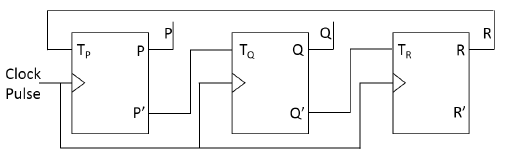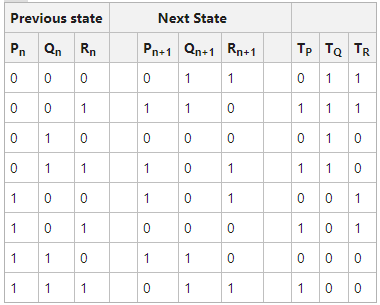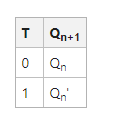# GATE | GATE CS 2021 | Set 1 | Question 38

• Difficulty Level : Hard
• Last Updated : 24 May, 2021

Consider a 3-bit counter, designed using T flip-flops, as shown below:Assuming the initial state of the counter given by PQR as 000, what are the next three states?
(A) 011,101,000
(B) 001,010,111
(C) 011,101,111
(D) 001,010,000

Explanation: Given Synchronous Sequential circuit (Same clock pulse applied ) has 3 bit T flip flop so using 3 bit we have 2^3=8 combinations. Given P(MSB) and R(LSB) lets construct Characteristics table.

Here TP=Rn , TQ=Pn’ , TQ=Qn’ .Using function table of T flip flop:We can obtain next state when T=0 previous state retains and when T=1 T flip flop toggles the previous state (i.e complement of previous state).

For example : TP=0 then Pn+1=0 as Pn=0, T=1 then Pn+1=1 as Pn=0.

PQR as initial state 000->011->101->000.

So next three states (000 as initial state ) will be 011,101,000.

Quiz of this Question

My Personal Notes arrow_drop_up
Recommended Articles
Page :